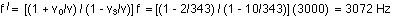## The Doppler effect

3-17-00

Sections 12.8 - 12.10

### The Doppler effect

The Doppler effect describes the shift in the frequency of a wave sound when the wave source and/or the receiver is moving. We'll discuss it as it pertains to sound waves, but the Doppler effect applies to any kind of wave. As with ultrasound, the Doppler effect has a variety of applications, ranging from medicine (with sound) to police radar and astronomy (with electromagnetic waves).

The Doppler effect is something you're familiar with. If you hear an emergency vehicle with its siren on, you notice an abrupt change in the frequency of the siren when it goes past you. If you are standing still when the vehicle is coming toward you, the frequency is higher than it would be if the vehicle was stationary; when the vehicle moves away from you, the frequency is lower. A similar effect occurs if the sound source is stationary and you move toward it or away from it.

At first glance you might think that there should be no difference between what happens when you move at a particular speed toward a source and when the source moves at the same speed toward you. As long as the speed is much less than the speed of sound, there is hardly any difference between these two cases. The higher the speeds involved, however, the greater the difference.

To convince yourself that it does make a difference which is moving, the source or the observer, consider what happens when v is equal to the speed of sound. When the receiver moves at the speed of sound toward the source, twice as many waves are intercepted as by a stationary observer, and the frequency is doubled. The waves are still nicely separated, however. On the other hand, when the source moves toward the receiver at the speed of sound, the sound waves pile up on top of each other (resulting in a sonic boom), and the frequency is effectively infinite.

Consider first the case of a stationary source, and an observer (you, for example) moving toward the source. As shown in the diagram, the waves are emitted by the source uniformly.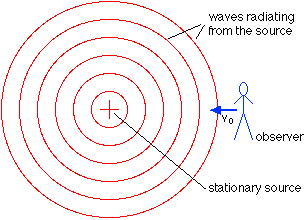If the observer is stationary, the frequency received by the observer is the frequency emitted by the source: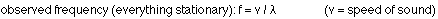If the observer moves toward the source at a speed vo, more waves are intercepted per second and the frequency received by the observer goes up. Effectively, the observer's motion shifts the speed at which the waves are received; it's basically a relative velocity problem. The observed frequency is given by: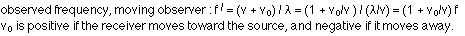If the observer is stationary but the source moves toward the observer at a speed vs, the observer still intercepts more waves per second and the frequency goes up.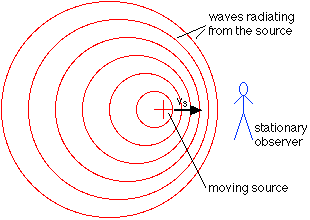This time it is the wavelength of the wave received by the observer that is effectively shifted by the motion, rather than the speed. The effective wavelength is simply:The frequency of waves received by the observer is then: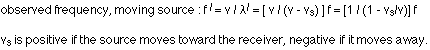In the most general case, in which both the source and receiver are moving, the observed frequency is: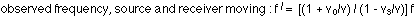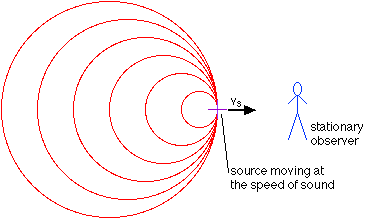Sonic booms occur when the source travels faster than the speed of sound. If the source is traveling at the speed of sound, the waves pile up and move along with the source; when the source travels faster than sound, a shock wave (also known as a sonic boom) occurs as waves pile up. The angle at which the shock wave moves away from the path of the source depends on the speed of the source relative to the speed of sound.### A Doppler example

A source is traveling east at 10 m/s toward you; you're traveling at 2 m/s east. It's 20°C. When the source is not moving it emits sound of frequency 3000 Hz. What frequency do you hear?

Sound in air at 20°C travels at 343 m/s. Plugging all of this information into the equation, and making sure we get the signs right, gives: